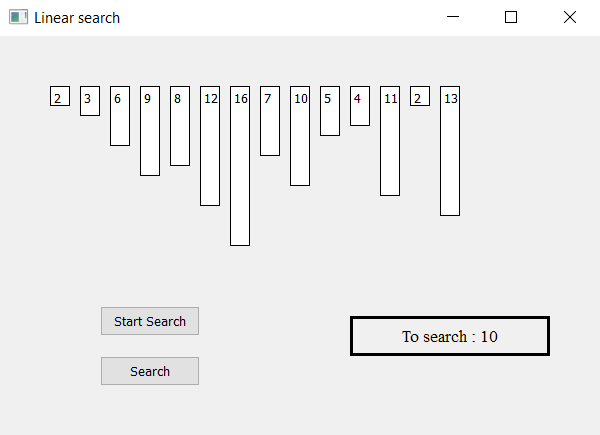Related Articles

# Linear Search Visualizer using PyQt5

• Last Updated : 06 May, 2020

In this article we will see how we can make a PyQt5 application which will visualize the linear search algorithm.

Linear search or sequential search is a method for finding an element within a list. It sequentially checks each element of the list until a match is found or the whole list has been searched.Attention geek! Strengthen your foundations with the Python Programming Foundation Course and learn the basics.

To begin with, your interview preparations Enhance your Data Structures concepts with the Python DS Course. And to begin with your Machine Learning Journey, join the Machine Learning - Basic Level Course

Gui implementation steps :

1. Create a list of label according to the given list of numbers
2. Set their text, border, color and geometry with the respective gap from each other
3. Each label height should be proportional to the value of each number
4. Create a start and pause push button to start the searching and pause the searching
5. Create a result label to show the searching status

Back end implementation steps :
1. Create label list corresponding to the given numbers
2. Create a variable to count the index and flag for searching
3. Add action to the push button their action should change the flag status i.e start action should make flag true and pause action should make flag false
4. Create a timer object which calls a method after every specific time
5. Inside the timer method check for the flag is flag is true begin the linear search algorithm
6. If label value if not equal make its color grey then check for next, if value got found make label color green and stop the search and show the output in the result label.
7. If the index counter becomes equal to the list length stop the search and show result as not found.

Below is the implementation

 `# importing libraries``from` `PyQt5.QtWidgets ``import` `*` `from` `PyQt5 ``import` `QtCore, QtGui``from` `PyQt5.QtGui ``import` `*` `from` `PyQt5.QtCore ``import` `*` `import` `sys`` ` ` ` `class` `Window(QMainWindow):`` ` `    ``# list of numbers``    ``number ``=` `[``2``, ``3``, ``6``, ``9``, ``8``, ``12``, ``16``, ``7``, ``10``, ``5``, ``4``, ``11``, ``2``, ``13``]``    ``def` `__init__(``self``):``        ``super``().__init__()`` ` `        ``# setting title``        ``self``.setWindowTitle(``"Linear search "``)`` ` `        ``# setting geometry``        ``self``.setGeometry(``100``, ``100``, ``600``, ``400``)`` ` `        ``# calling method``        ``self``.UiComponents()`` ` `        ``# showing all the widgets``        ``self``.show()`` ` `    ``# method for widgets``    ``def` `UiComponents(``self``):`` ` `        ``# start flag``        ``self``.start ``=` `False`` ` `        ``# list to hold labels``        ``self``.label_list ``=` `[]`` ` `        ``# desired value``        ``self``.desired ``=` `11`` ` `        ``# counter for keeping index``        ``self``.counter ``=` `0`` ` `        ``# local counter``        ``c ``=` `0`` ` `        ``# iterating list of numbers``        ``for` `i ``in` `self``.number:`` ` `            ``# creating label for each number``            ``label ``=` `QLabel(``str``(i), ``self``)`` ` `            ``# adding background color and border``            ``label.setStyleSheet("border : ``1px` `solid black;``                                 ``background : white;")`` ` `            ``# aligning the text``            ``label.setAlignment(Qt.AlignTop)`` ` `            ``# setting geometry using local counter``            ``# first parameter is distance from left ``            ``# and second is distance from top``            ``# third is width and forth is height``            ``label.setGeometry(``50` `+` `c ``*` `30``, ``50``, ``20``, i ``*` `10` `+` `10``)`` ` `            ``# adding label to the label list``            ``self``.label_list.append(label)`` ` `            ``# incrementing local counter``            ``c ``=` `c ``+` `1`` ` ` ` `        ``# creating push button to start the search``        ``self``.search_button ``=` `QPushButton(``"Start Search"``, ``self``)`` ` `        ``# setting geometry of the button``        ``self``.search_button.setGeometry(``100``, ``270``, ``100``, ``30``)`` ` `        ``# adding action to the search button``        ``self``.search_button.clicked.connect(``self``.search_action)`` ` `        ``# creating push button to pause the search``        ``pause_button ``=` `QPushButton(``"Pause"``, ``self``)`` ` `        ``# setting geometry of the button``        ``pause_button.setGeometry(``100``, ``320``, ``100``, ``30``)`` ` `        ``# adding action to the search button``        ``pause_button.clicked.connect(``self``.pause_action)`` ` `        ``# creating label to show the result``        ``self``.result ``=` `QLabel(``"To search : "` `+` `str``(``self``.desired), ``self``)`` ` `        ``# setting geometry``        ``self``.result.setGeometry(``350``, ``280``, ``200``, ``40``)`` ` `        ``# setting style sheet``        ``self``.result.setStyleSheet(``"border : 3px solid black;"``)`` ` `        ``# adding font``        ``self``.result.setFont(QFont(``'Times'``, ``10``))`` ` `        ``# setting alignment``        ``self``.result.setAlignment(Qt.AlignCenter)`` ` `        ``# creating a timer object``        ``timer ``=` `QTimer(``self``)`` ` `        ``# adding action to timer``        ``timer.timeout.connect(``self``.showTime)`` ` `        ``# update the timer every 200 millisecond``        ``timer.start(``200``)`` ` `    ``# method called by timer``    ``def` `showTime(``self``):`` ` `        ``# checking if flag is true``        ``if` `self``.start:``            ``# linear search`` ` `            ``# checking if the element is equal to desired element``            ``if` `self``.label_list[``self``.counter].text() ``=``=` `str``(``self``.desired):`` ` `                ``# make its color green``                ``self``.label_list[``self``.counter].setStyleSheet(``                                  ``"border : ``1px` `solid black; ``                                   ``background : lightgreen;")`` ` `                ``# show result in result label``                ``self``.result.setText(``"Found at index : "``                                     ``+` `str``(``self``.counter))`` ` `                ``# make the start flag false``                ``self``.start ``=` `False`` ` `                ``# reseting the counter``                ``self``.counter ``=` `0`` ` `            ``# if element is not equal``            ``else``:`` ` `                ``# make the label color grey``                ``self``.label_list[``self``.counter].setStyleSheet(``                                  ``"border : ``1px` `solid black;``                                   ``background : grey;")`` ` `            ``# increment the counter``            ``self``.counter ``+``=` `1`` ` `            ``# if counter value become equal to list length``            ``if` `self``.counter ``=``=` `len``(``self``.label_list):`` ` `                ``# make start flag false``                ``self``.start ``=` `False`` ` `                ``# show result i.e not found``                ``self``.result.setText(``"Not Found"``)`` ` `    ``# method called by search button``    ``def` `search_action(``self``):`` ` `        ``# making flag true``        ``self``.start ``=` `True`` ` `        ``# showing text in result label``        ``self``.result.setText(``"Started searching..."``)`` ` `    ``# method called by pause button``    ``def` `pause_action(``self``):`` ` `        ``# making flag false``        ``self``.start ``=` `False`` ` `        ``# showing text in result label``        ``self``.result.setText(``"Paused"``)`` ` ` ` `# create pyqt5 app``App ``=` `QApplication(sys.argv)`` ` `# create the instance of our Window``window ``=` `Window()`` ` `# start the app``sys.exit(App.``exec``())`

Output :

My Personal Notes arrow_drop_up